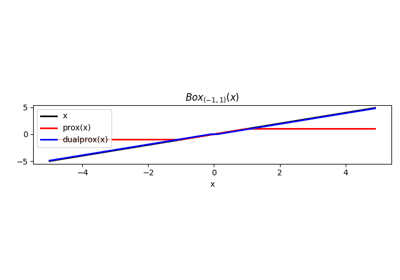# pyproximal.Simplex#

pyproximal.Simplex(n, radius, dims=None, axis=- 1, maxiter=100, ftol=1e-08, xtol=1e-08, call=True, engine='numpy')[source]#

Simplex proximal operator.

Proximal operator of a Simplex: $$\Delta_n(r) = \{ \mathbf{x}: \sum_i x_i = r,\; x_i \geq 0 \}$$. This operator can be applied to a single vector as well as repeatedly to a set of vectors which are defined as the rows (or columns) of a matrix obtained by reshaping the input vector as defined by the dims and axis parameters.

Parameters
nint

Number of elements of input vector

radiusfloat

dimstuple, optional

Dimensions of the matrix onto which the input vector is reshaped

axisint, optional

Axis along which simplex is repeatedly applied when dims is not provided

maxiterint, optional

Maximum number of iterations used by bisection

ftolfloat, optional

Function tolerance in bisection (only with engine='numba' or engine='cuda')

xtolfloat, optional

Solution absolute tolerance in bisection

callbool, optional

Evalutate call method (True) or not (False)

enginestr, optional

Engine used for simplex computation (numpy, numbaor cuda).

Raises
KeyError

If engine is neither numpy nor numba nor cuda

ValueError

If dims is provided as a list (or tuple) with more or less than 2 elements

Notes

As the Simplex is an indicator function, the proximal operator corresponds to its orthogonal projection (see pyprox.projection.SimplexProj for details.

Note that tau does not have effect for this proximal operator, any positive number can be provided.

## Examples using pyproximal.Simplex#Norms

Norms